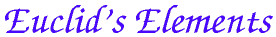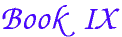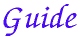Ok

En poursuivant votre navigation sur ce site, vous acceptez l'utilisation de cookies. Ces derniers assurent le bon fonctionnement de nos services. En savoir plus.

##Proposition 36

 If as many numbers as we please beginning from a unit are set out continuously in double proportion until the sum of all becomes prime, and if the sum multiplied into the last makes some number, then the product is perfect. Let as many numbers as we please, A, B, C, and D, beginning from a unit be set out in double proportion, until the sum of all becomes prime, let E equal the sum, and let E multiplied by D makeFG. I say that FG is perfect. For, however many A, B, C, and D are in multitude, take so many E, HK, L, and M in double proportion beginning from E. Therefore, ex aequali A is to D as E is to M. Therefore the product of E and D equals the product of A and M. And the product of E and D is FG, therefore the product of A and M is also FG. VII.14 VII.19 Therefore A multiplied by M makes FG. Therefore M measures FG according to the units in A. And A is a dyad, therefore FG is double of M. But M, L, HK, and E are continuously double of each other, therefore E, HK, L, M, and FG are continuously proportional in double proportion. Subtract from the second HK and the last FG the numbers HN and FO, each equal to the first E. Therefore the excess of the second is to the first as the excess of the last is to the sum of those before it. Therefore NK is to E as OG is to the sum of M, L, KH, and E. IX.35 And NK equals E, therefore OG also equals M, L, HK, E. But FO also equals E, and E equals the sum of A, B, C, D and the unit. Therefore the whole FG equals the sum of E, HK, L, M, A, B, C, D, and the unit, and it is measured by them. I say also that FG is not measured by any other number except A, B, C, D, E, HK, L, M, and the unit. If possible, let some number P measure FG, and let P not be the same with any of the numbers A, B, C, D, E, HK, L, or M. And, as many times as P measures FG, so many units let there be in Q, therefore Q multiplied by P makes FG. But, further, E multiplied by D makes FG, therefore E is to Q as P is to D. VII.19 And, since A, B, C, and D are continuously proportional beginning from a unit, therefore D is not measured by any other number except A, B, or C. IX.13 And, by hypothesis, P is not the same with any of the numbers A, B, or C, therefore P does not measure D. But P is to D as E is to Q, therefore neither does E measure Q. VII.Def.20 And E is prime, and any prime number is prime to any number which it does not measure. Therefore E and Q are relatively prime. VII.29 But primes are also least, and the least numbers measure those which have the same ratio the same number of times, the antecedent the antecedent and the consequent the consequent, and E is toQ as P is to D, therefore E measures P the same number of times that Q measures D. VII.21 VII.20 But D is not measured by any other number except A, B, or C, therefore Q is the same with one of the numbers A, B, or C. Let it be the same with B. And, however many B, C, and D are in multitude, take so many E, HK, and L beginning from E. Now E, HK, and L are in the same ratio with B, C, and D, therefore, ex aequali B is to D as E is to L. VII.14 Therefore the product of B and L equals the product of D and E. But the product of D and E equals the product of Q and P, therefore the product of Q and P also equals the product of B and L. VII.19 Therefore Q is to B as L is to P. And Q is the same with B, therefore L is also the same with P, which is impossible, for by hypothesis P is not the same with any of the numbers set out. VII.19 Therefore no number measures FG except A, B, C, D, E, HK, L, M, and the unit. And FG was proved equal to the sum of A, B, C, D, E, HK, L, M, and the unit, and a perfect number is that which equals its own parts, therefore FG is perfect. VII.Def.22 Therefore, if as many numbers as we please beginning from a unit are set out continuously in double proportion until the sum of all becomes prime, and if the sum multiplied into the last makes some number, then the product is perfect. Q.E.D.

####### Summary of the proof

Euclid begins by assuming that the sum of a number of powers of 2 (the sum beginning with 1) is a prime number. Let p be the number of powers of 2, and let s be their sum which is prime.s = 1 + 2 + 22 + ... + 2p-1

Note that the last power of 2 is 2p-1 since the sum starts with 1, which is 20.

In Euclid's proof, A represents 2, B represents 22C represents 23, and D is supposed to be the last power of 2, so it represents 2p-1. Also, E represents their sum s, and FG is the product of E and D, so it represents s2p-1. Let's denote that last by n.

n = s2p-1

The goal is to show that n is a perfect number.

In the first part of this proof, Euclid finds some proper divisors of n that sum to n. These come in two sequences:

1, 2, 22, ..., 2p-1ands, 2s, 22s, ..., 2n-2s

In his proof, the latter are represented by E, HK, L, and finally M.

It is clear that each of these is a proper divisor of n, and later in the proof Euclid shows that they are the only proper divisors of n.

Using the previous proposition, IX.35, Euclid finds the sum of the continued proportion,

s + 2s + 22s + ... + 2n-2s,

to be 2n-1s – s. But s was the sum 1 + 2 + 22 + ... + 2p-1, hence,

 n = 2n-1s = 1 + 2 + 22 + ... + 2p-1 + s + 2s + 22s + ... + 2n-2s

Thus, n is a sum of these proper divisors.

All that is left to do is to show that they are the only proper divisors of n, for then n will be the sum of all of its proper divisors, whence a perfect number.

The remainder of the proof is detailed and difficult to follow. It hinges on IX.13 which implies that the only factors of 2p-1 are powers of 2, so all the factors of 2p-1 have been found. Here's a not-too-faithful version of Euclid's argument. Suppose n factors as ab where a is not a proper divisor of n in the list above. In Euclid's proof, P represents a and Q represents b.

Since a divides s 2p-1, but is not a power of 2, and s is prime, therefore s divides a. Then b has to be a power of 2. But then a has to be a power of 2 times s. But all the powers of 2 times s are on the list of known proper divisors. Therefore, the list includes all the proper divisors.

#### Mersenne primes and perfect numbers

Note that the sum, s = 1 + 2 + 22 + ... + 2p-1, equals 2p – 1, by IX.35. As this fact is not needed in the proof, Euclid omits to mention it. Thus, we can restate the proposition as follows:
If 2p – 1 is a prime number, then (2p – 1) 2p-1 is a perfect number.
Prime numbers of the form 2p – 1 have come to be called Mersenne primes named in honor of Marin Mersenne (1588-1648), one of many people who have studied these numbers. The four smallest perfect numbers, 6, 28, 496, and 8128, were known to the ancient Greek mathematicians. The Mersenne primes 2p – 1 corresponding to these four perfect numbers are 3, 7, 31, and 127, respectively, where the exponents p are 2, 3, 5, and 7, respectively.

The observation that these four exponents are all prime suggests the following two questions:

1. In order for 2p – 1 to be prime, is it sufficient for p to be prime?
2. In order for 2p – 1 to be prime, is it necessary for p to be prime?
Naturally, the next number to check for primality is 211 – 1, 2047, which, by a simple search for prime factors is found not to be prime. The number 2047 factors as 23 times 89. Therefore, primality of p isnot sufficient.

In 1640 Pierre de Fermat (1601-1665) wrote to Mersenne with his investigation of these primes. Fermat found three conditions on p that were necessary for 2p – 1 to be prime. One of these conditions answers the second question above— p does have to be prime. Here's a quick argument for that. If p did factor, say as ab, then 2p – 1, which is 2ab – 1, would also factor, namely as

2ab – 1 = (2a – 1) (2a(b-1) + 2a(b-2)  + ... + 2a).

Many mathematicians have studied Mersenne primes since then. A fairly practical testing algorithm was constructed by Lucas in 1876. He showed that the the number 2p – 1 is prime if and only if it divides the number S(p-1), where S(p-1) is defined recursively: S(1) = 4, and S(n+1) = S(n)2 – 2.

The search for more Mersenne primes, and therefore more perfect numbers, continues. It is not known if there are infinitely many or finitely many even perfect numbers. Mersenne primes are scarce, but more continue to be found. There are at least 39 of them, the largest known (as of December 2005) is 230402457 – 1. It has 9152052 digits. For more information, see the The Great Internet Mersenne Prime Search, GIMPS.

There is a also a question about odd perfect numbers: Are there any? It has been shown that there are no small odd perfect numbers; it is known that odd numbers with fewer then 300 digits are not perfect. It may well be that there are no odd perfect numbers, but to date there is no proof.

Next book: Book X introduction

Previous proposition: IX.35

Book IX introduction

 Select from Book IX    Book IX intro    IX.1    IX.2    IX.3    IX.4    IX.5    IX.6    IX.7    IX.8    IX.9    IX.10    IX.11    IX.12    IX.13    IX.14    IX.15    IX.16    IX.17    IX.18    IX.19    IX.20    IX.21    IX.22    IX.23    IX.24    IX.25    IX.26    IX.27    IX.27    IX.28    IX.29    IX.30    IX.31    IX.32    IX.33    IX.34    IX.35    IX.36 Select book    Book I    Book II    Book III    Book IV    Book V    Book VI    Book VII    Book VIII    Book IX    Book X    Book XI    Book XII    Book XIII Select topic    Introduction    Table of Contents    Geometry applet    About the text    Euclid    Web references    A quick trip